## 梯度下降法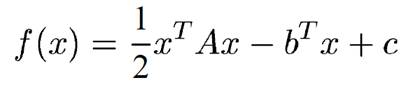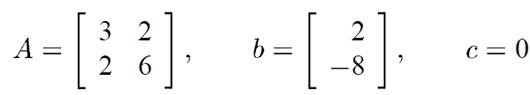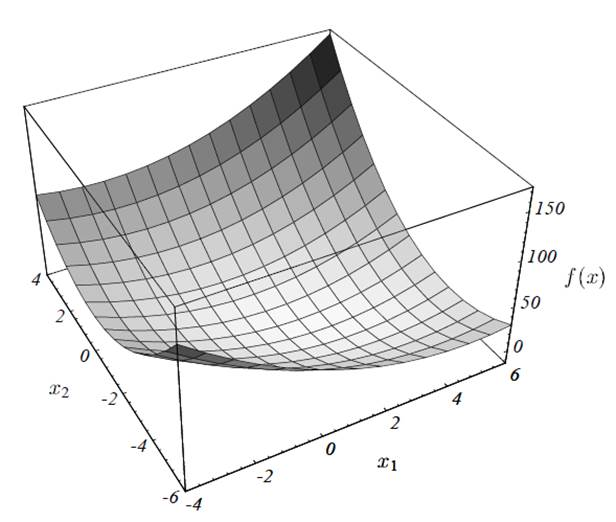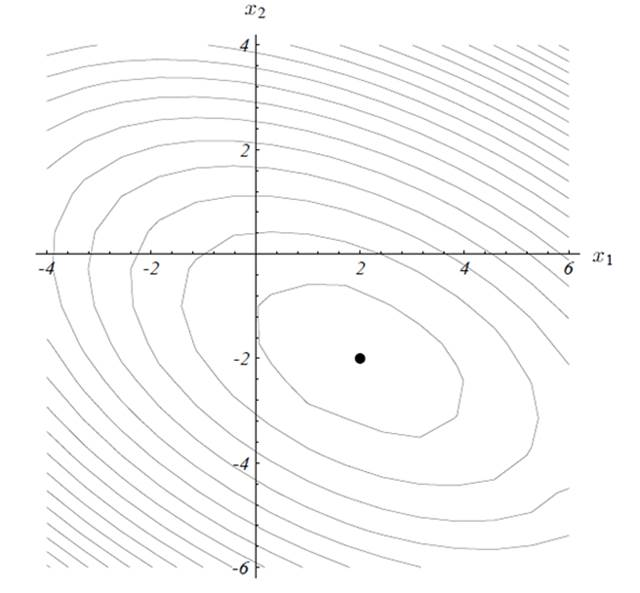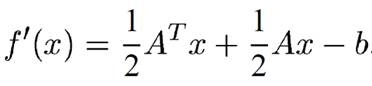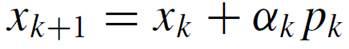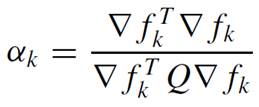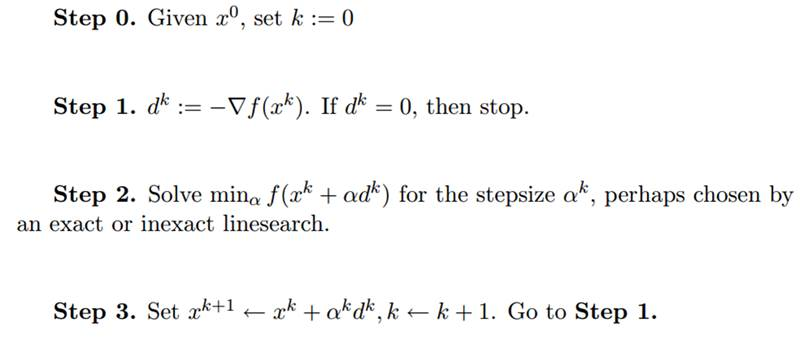## 梯度下降法的实现

 1 2 3 4 5 6 7 8  Struct Problem{ FUNC f_; GRAD grad_; Vec x0_; double epsilon_; int maxiterate_; STEPFUNC step_; } 

  1 2 3 4 5 6 7 8 9 10 11 12 13 14 15 16 17 18 19 20 21 22 23  class Desent { public: Vec operator()(const Problem& p)const { Vec x = p.x0_; for (int i = 0; i < p.maxiterate_; i++) { Vec g = p.grad_(x); if (g.norm() <= p.epsilon_) break; // converge Vec d = this->dir(p, x); double steplength = p.step_(x, g, d); x += steplength * d; if (postproc) postproc(x); } return x; } std::function&)> postproc; protected: virtual Vec dir(const Problem& p, const Vec& x) const= 0; }; 

  1 2 3 4 5 6 7 8 9 10  class SteepestDesent : public Desent{ public: protected: virtual Vec dir(const Problem& p, const Vec& x)const { return -p.grad_(x); } }; 

## 参考文献

 Nocedal J, Wright S. Numerical optimization[M]. Springer Science & Business Media, 2006.

 Shewchuk J R. An introduction to the conjugate gradient method without the agonizing pain[J]. 1994.

袁亚湘, 孙文瑜. 最优化理论和方法[J]. 1997.